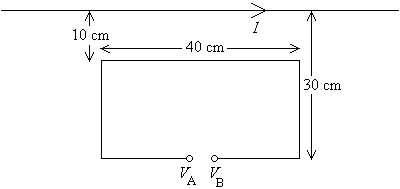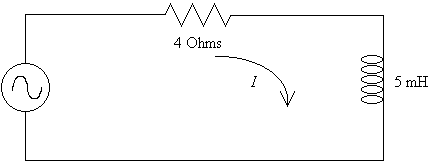First Name: ___________________ Last Name: ____________________ Section: _________

March 26, 1999 Physics 208

Exam 2

Print your name and section clearly on all five pages. (If you do not know your section number, write your TAs name.) Show all work in the space immediately below each problem. Your final answer must be placed in the box provided. Problems will be graded on reasoning and intermediate steps as well as on the final answer. Be sure to include units wherever necessary, and the direction of vectors. Each problem is worth 25 points. In doing the problems, try to be neat. Check your answers to see that they have the correct dimensions (units) and are the right order of magnitudes. You are allowed one 8½ x 11" sheet of notes and no other references. The exam lasts exactly 50 minutes.

(Do not write below)

SCORE:

Problem 1: __________

Problem 2: __________

Problem 3: __________

Problem 4: __________

TOTAL: ___________

First Name: ___________________ Last Name: ____________________ Section: _________

1. A long, straight wire carries a current I that starts at zero at time t = 0 and increases at a rate of 1 A/ms thereafter.a. What is the magnetic field 20 cm from the wire at t = 50 ms? (8 pts.)

b. What is the magnetic flux at t = 50 ms through the loop shown? (9 pts.)

c. What is the voltage VB  VA? (8 pts.)

First Name: ___________________ Last Name: ____________________ Section: _________

2. In the circuit below the voltage source is sinusoidal with a magnitude of 20 V and a frequency of 60 Hz.a. What is the magnitude of the current I in the circuit? (9 pts.)

b. What is the phase of the current relative to the source voltage? (8 pts.)

c. What average power does the voltage source supply? (8 pts.)

First Name: ___________________ Last Name: ____________________ Section: _________

3. When the sun is directly overhead, its intensity is about 1500 W/m2 at the surface of the earth.

a. What is the rms electric field in this sunlight? (6 pts.)

b. What is the rms magnetic field in this sunlight? (6 pts.)

c. Assuming the average photon has a wavelength of 600 nm, how many photons strike a 1 m2 area directly below the sun each second? (6 pts.)

d. Assuming the sunlight is half absorbed and half reflected by the earth (R = 6370 km), what total radiation force is exerted on the earth? (7 pts.)

First Name: ___________________ Last Name: ____________________ Section: _________

4. An object with a height of 2 cm is placed 10 cm in front of a converging lens with a focal length of 20 cm.

a. Where is the image located? (6 pts.)

b. What is the height of the image? (6 pts.)

c. Is the image real or virtual, upright or inverted? (6 pts.)

d. If the lens is thin with an index of refraction of 1.33 and equally curved on both faces, what is the radius of curvature of the faces? (7 pts.)